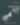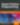# Learning Loops

PHP

Share:

#### Free JavaScript Book!Write powerful, clean and maintainable JavaScript.

RRP \$11.95

A significant advantage of computers is that they can perform repetitive tasks easily and efficiently. Instead of writing repetitive code you can write a set of statements that processes some data and then have the computer execute them repeatedly by using a construct known as a loop. Loops come in several different flavors in PHP: `for`, `while`, `do-while`, and `foreach`. I’ll introduce you to each of them and show you how they can making repetitive tasks straightforward and easy to maintain.

## The `for` Loop

Let’s consider the example of generating a multiplication table for the number 12. You could calculate and display the values resulting from multiplying 12 by 1, 2, 3 etc. like so:

``````<?php
echo "1 × 12 = " . (1 * 12) . "<br>";
echo "2 × 12 = " . (2 * 12) . "<br>";
echo "3 × 12 = " . (3 * 12) . "<br>";
...``````

… and so on. Maybe extending the code to 12×12 would be quick, a simple matter of copy, paste, and amend. But what if the calculation needed to go up to 50×12? The copy and paste approach is really an inefficient use of your time and can lead to unwieldy code down the road.

But never fear! A solution is at hand – the `for` loop.

``````<?php
for (\$i = 1; \$i <= 12; \$i++) {
echo \$i . " × 12 = " . (\$i * 12) . "<br>";
}``````

This example shows how few lines are needed to create the multiplication table for any arbitrary value.

There are three separate expressions used with a for loop which are separated by semicolons and contained within the parentheses:

• The first expression `\$i = 1` initializes the variable `\$i` to be used as a counter (`\$i` is a commonly used variable name for loop counters). The counter will be used to keep track of how many times the loop has executed.
• The second expression `\$i <= 12` determines whether to stop the loop or proceed. In this case, the loop will execute 12 times. To be more precise the loop will continue while `\$i` has a value less than or equal to 12. Once `\$i` has a higher value, the loop will stop.
• The third expression `\$i++` updates the counter. `\$i` is incremented by 1 each time after the loop as completed a cycle. A cycle of a loop is also known as an iteration.

From a maintenance perspective, if you need to change the code to extend the range up to 50×12, then only the value in the second expression needs to be changed:

``````<?php
for (\$i = 1; \$i <= 50; \$i++) {
echo \$i . " × 12 = " . (\$i * 12) . "<br>";
}``````

This is clearly better than adding 38 more lines of code!

Now what if you want to process in reverse i.e. multiplying 12 by 12, then 11, then 10, and so on? It’s easy if you simply adjust the three expressions.

``````<?php
for (\$i = 12; \$i > 0; \$i--) {
echo \$i . " × 12 = " . (\$i * 12) . "<br>";
}``````

`\$i` is initialized to 12, the value the loop needs to start with. `\$i > 0` ensures the loop will only iterate while `\$i` is 1 or greater. After each cycle, the value of `\$i` is decremented by one.

## The `while` and `do-while` Loops

A `for` loop is suitable when you have to carry out a finite number of actions. There may be times though when you need to carry out repetitive actions for an unspecified number of iterations. In this circumstance either of the while or `do-while` loops is ideal.

This example illustrates a `do-while` loop that reads a file and prints the contents – you don’t know in advance how many lines of data there are in this file so using for would clearly not be suitable.

``````<?php
\$file = "employees.csv";
\$employeeFile = fopen(\$file, "r");
do {
\$data = fgets(\$employeeFile);
echo \$data . "<br>";
}
while (!feof(\$employeeFile));``````

Note that the `do` keyword precedes the opening brace and the `while` clause follows the closing brace. The loop will continue until reaching the end of the file, irrespective of whether there are 5 or 500 lines.

The same example as a while loop only looks like this:

``````<?php
\$file = "employees.csv";
\$employeeFile = fopen(\$file, "r");
while (!feof(\$employeeFile)) {
\$data = fgets(\$employeeFile);
echo \$data . "<br>";
}``````

So how does this differ from `do-while`? There is a subtle but important difference – `do-while` will execute at least once because the end of file condition is checked only after the first iteration. The `while` loop begins execution only if we are not at the end of file.

## The `foreach` Loop

You’ve seen a straightforward example of printing the multiplication table for the number 12. Let’s look now at another example, this time using an array. As the article Introduction to PHP Arrays points out, arrays are essentially indexed tables of data. Consider this array holding the months of the year:

==img1==

To access the value, you need the associated key. To illustrate how you might use `\$i` as a key as well as a counter, let’s print calendar months from an array called `\$months`:

``````<?php
\$months = array("January", "February", "March", ... "Dec");
for (\$i = 0; \$i < 12; \$i++) {
echo \$months[\$i] . "<br>";
}``````

You are using the `for` loop like before but with two subtle differences – first, `\$i` is initialized to 0 because the first item in an automatically-indexed array is 0. Second, the condition checks that the counter is less than 12 because the loop must stop once 12 items have been processed (key values 0 to 11 in the array).

You may have guessed there is an alternative to using for. Yes, that alternative is `foreach`. It doesn’t require you to know in advance how many array elements there are or to keep track of a counter variable.

There are two variations of the `foreach` loop and I’ll show you both. The first retrieves just the value of each array element.

``````<?php
foreach (\$months as \$value) {
echo \$value . "<br>";
}``````

You can see how this is a simpler solution for iterating through an array than using `for`. Within the parentheses you provide the array name followed by the `as` keyword and a variable to which will be assigned the current element’s value. In this case, `\$months` is the array and `\$value` is the value variable. There is no need for counters or checking whether the loop should continue and, if the array size changes, there is no need to change any code.

In the other `foreach` variant you have access to the key as well as the value:

``````<?php
foreach (\$months as \$key => \$mon) {
echo \$key . " - " . \$mon . "<br>";
}``````

Here `\$key =>` has been added to the expression, which allows you to access the key value.

## The `break` and `continue` Keywords

I’ve assumed throughout this article that you want to process a loop from start to finish. It is of course possible that you may want to exit from a loop early if a certain condition occurs. For this you can use the `break` keyword, as shown in the following modified example of the earlier `do-while` loop:

``````<?php
\$file = "employee.sql";
\$employeeFile = fopen(\$file, "r");
do {
\$data = fgets(\$employeeFile);
if (strlen(\$data) == 0) {
break;
}
else {
echo \$data;
}
}
while (!feof(\$employeeFile));``````

Each line of the file is retrieved and checked – if the line is blank, `break` is used to exit the loop immediately. The `while` clause keeps the loop running as long as it has not yet reached end of the file.

If you do not want to exit from the loop, but instead carry on with the next iteration, use the `continue` keyword instead.

## Summary

In this article you’ve seen there are a variety of ways in which loops can be used for repetitive tasks and to make your code easier to maintain. I’d like to leave you with some tips on using loops:

• Use `for` when you have a finite and known number of iterations.
• Use `while` or `do-while` when the number of iterations is not known but can be controlled by a condition (e.g. reaching the end of a file).
• Use `foreach` when you need to process each element in an array.
• If you need to break out completely from a loop, you can use `break`.
• If you want to skip the remaining statements for the iteration and continue on with the next one, you can use `continue`.

Image via Marcio Jose Bastos Silva / Shutterstock### Iain Tench

Iain Tench has worked in the IT industry for 30 years as a programmer, project manager (Prince2 Practitioner), consultant, and teacher. As a project manager, he specialized in integration projects mainly involving payment systems in the financial sector. He now teaches and has acquired a Masters degree in Internet Systems Development as well as a teaching qualification. Iain's specialized areas of teaching is web technologies, mainly programming in a variety of languages, HTML, CSS and database integration. He's also written a book about Dreamweaver CS3 for Hodder Education.

#### New books out now!Learn how Git works, and how to use it to streamline your workflow!Google, Netflix and ILM are Python users. Maybe you should too?